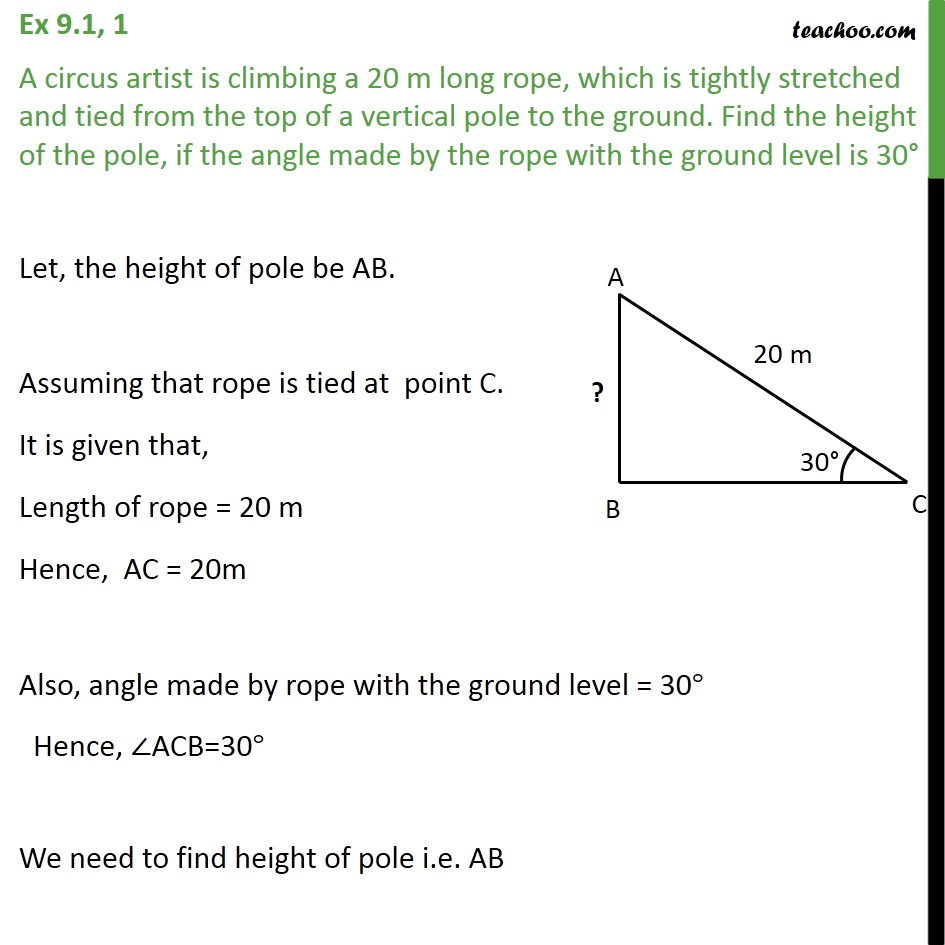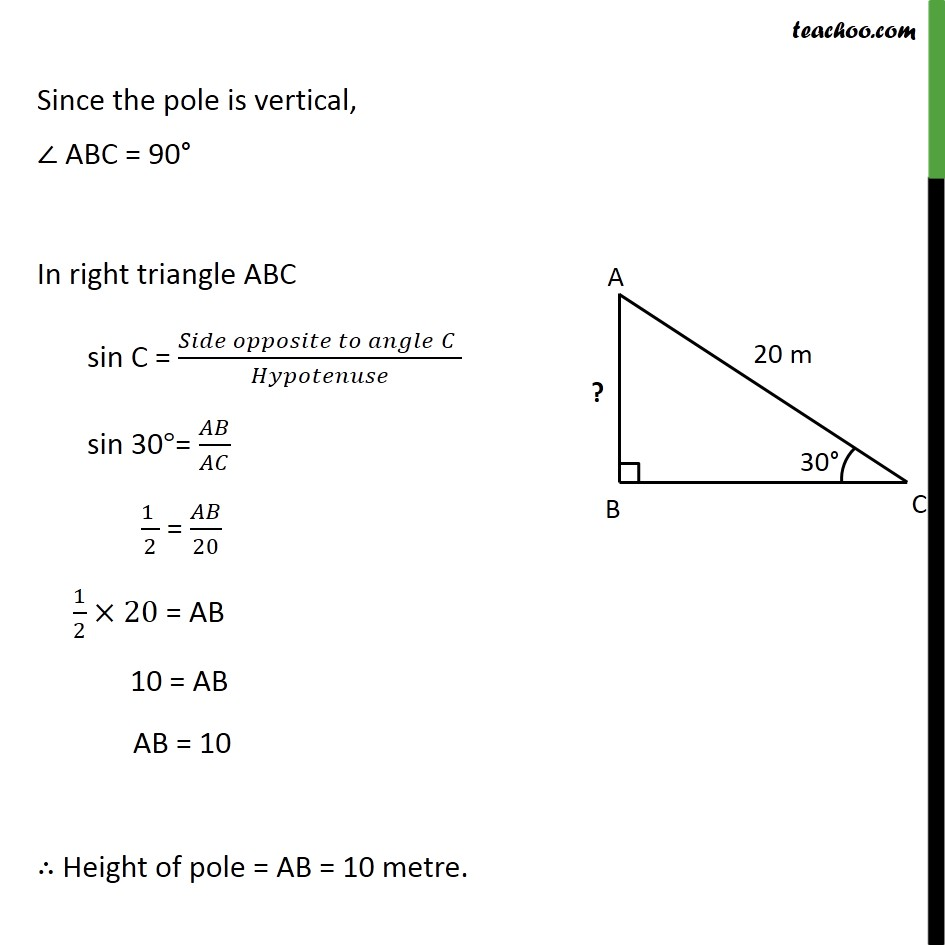1. Chapter 9 Class 10 Some Applications of Trigonometry (Term 2)
2. Concept wise
3. Questions easy to difficult

Transcript

Ex 9.1, 1 A circus artist is climbing a 20 m long rope, which is tightly stretched and tied from the top of a vertical pole to the ground. Find the height of the pole, if the angle made by the rope with the ground level is 30° Let, the height of pole be AB. Assuming that rope is tied at point C. It is given that, Length of rope = 20 m Hence, AC = 20m Also, angle made by rope with the ground level = 30° Hence, ∠ACB=30° We need to find height of pole i.e. AB Since the pole is vertical, ∠ ABC = 90° In right triangle ABC sin C = (𝑆𝑖𝑑𝑒 𝑜𝑝𝑝𝑜𝑠𝑖𝑡𝑒 𝑡𝑜 𝑎𝑛𝑔𝑙𝑒" " 𝐶" " )/𝐻𝑦𝑝𝑜𝑡𝑒𝑛𝑢𝑠𝑒 sin 30°= 𝐴𝐵/𝐴𝐶 (1 )/2 = 𝐴𝐵/20 1/2×20 = AB 10 = AB AB = 10 ∴ Height of pole = AB = 10 metre.